Rayleigh Quotient Iteration

These images are variants on some of the images that appeared in  and readers interested in the full details should consult that reference. The algorithm is explained in many numerical analysis texts and in . In summary, the Rayleigh Quotient algorithm is a method for estimating the eigenvalues and eigenvectors of a matrix M. We view it as beginning with a guess for the eigenvalue, u0 and the eigenvector x0. The eigenvalue estimate is improved by solving the linear system (M-u0 I) x1 = x0 where I is the identity matrix. The eigenvalue estimate is improved by u1=(x1HM x1)/(x1Hx1) . The process is iterated until an eigenvalue is found (the system is singular), or a maximum iteration count is reached. In practice, eigenvalues are obtained to high accuracy. The difficulty is in globally determining which eigenvalue is obtained. Our images show the basins of attraction of the eigenvalues. Each image coresponds to x0=1 1 1 or x0=1 1 1 1 according to the size of the matrix and uses various choices for matrix and region of the complex plane for the guess u0.

 `````` m2 m3 m6 m8 1 1 1 1 1 1 1 2 3 4 12 _14 0 4 9 8 4 9+i 8 4 2 5 6 7 7 _6 _2 4 5 6 2 5 6 2 3 6 8 9 _10 12 _2 _2 4 7 9 10 _18 22 _3 _4 ``````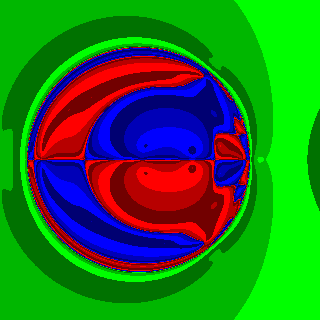Basins of attraction for m2 with Re(u0) in [ _15, 9]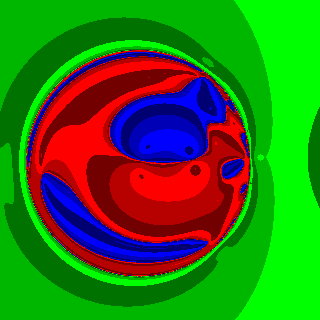Basins of attraction for m3 with Re(u0) in [ _15, 9]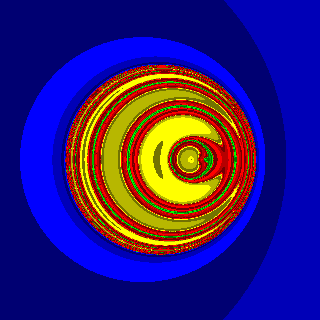Basins of attraction for m6 with Re(u0) in [ _20, 12]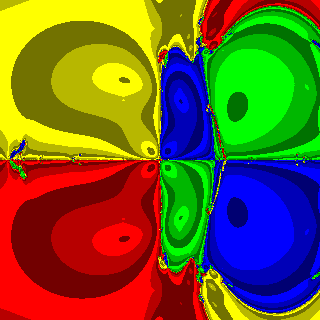Basins of attraction for m8 with Re(u0) in [ _20, 20]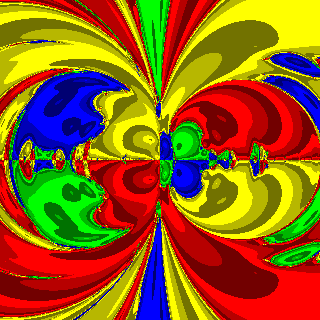Basins of attraction for m8 with Re(u0) in [ _80, 80]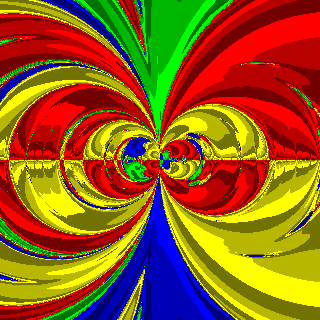Basins of attraction for m8 with Re(u0) in [ _320, 320]
If one forgets to compute the conjugates required in u1=(x1HM x1)/(x1Hx1) and instead computes u1=(x1TM x1)/(x1Tx1), the following interesting images occur. Black correspnds to points where an eigenvalue was not found before the maximum iteration count occurred.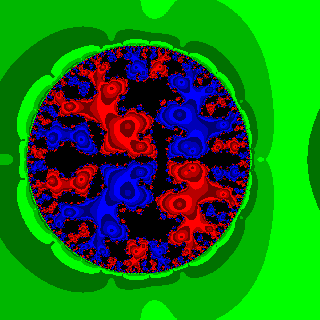Basins of attraction for m2 with Re(u0) in [ _15, 9]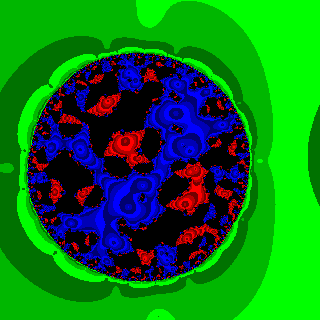Basins of attraction for m3 with Re(u0) in [ _15, 9]
 Clifford A. Reiter, Visualizing the Dynamics of the Rayleigh Quotient Iteration, Computers & Graphics, 16 3 (1992) 341-344.
 Clifford Reiter, Easy Algorithms for Finding Eigenvalues, Mathematics Magazine, 63 3 (1990), 173-178.
 Cliff Reiter, Sample J6.01 script for creating Rayleigh quotient iteration images. See notes at the top for other requirements. Out of date scripts: for J4.06 for J5.04 .